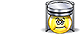Unit Conversion Software Web Widgets Loan Calculator Currency Rates Country Flags Unit Converter
 Faq Help# Torr ConversionContact

## Torr, unit of measure

The torr (symbol: Torr) or millimeter of mercury (mmHg) is a non-SI unit of pressure. It is the atmospheric pressure that supports a column of mercury 1 millimeter high. The unit is named after Evangelista Torricelli, Italian physicist and mathematician, for his discovery of the principle of the barometer in 1643. A related unit is the micrometre of mercury (µmHg), which is one thousand times smaller.
To perform conversions between torr and other Pressure units please try our Pressure Unit Converter

Convert torr to:
pascal, exapascal, petapascal, terapascal, gigapascal, megapascal, kilopascal, hectopascal, dekapascal, decipascal, centipascal, millipascal, micropascal, nanopascal, picopascal, femtopascal, attopascal, newton/square meter, newton/square centimeter, newton/square millimeter, kilonewton/square meter, bar, millibar, microbar, dyne/square centimeter, kilogram-force/square meter, kilogram-force/square centimeter, kilogram-force/square millimeter, gram-force/square centimeter, ton-force (short)/square foot, ton-force (short)/square inch, ton-force (long)/square foot, ton-force (long)/square inch, kip-force/square inch, ksi, pound-force/square foot, pound-force/square inch, psi, poundal/square foot, centimeter mercury (0°C), millimeter mercury (0°C), inch mercury (32°F), inch mercury (60°F), centimeter water (4°C), millimeter water (4°C), inch water (4°C), foot water (4°C), inch water (60°F), foot water (60°F), atmosphere technical, Standard atmosphereDownload Pressure Unit Converter
our powerful software utility that helps you make easy conversion between more than 2,100 various units of measure in more than 70 categories. Discover a universal assistant for all of your unit conversion needs - download the free demo version right away!Copyright © 1998-2009 UnitConversion.org Privacy & Terms | About | Faq | Help | Contact | Link to Us